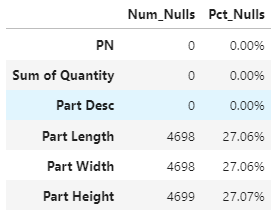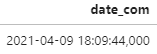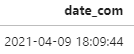APP推广合作

Pandas数据清理，看这一篇就够了
2021-08-11 11:14:46Source: Quora

https://github.com/ClaireWithGithub/pandas_data_cleaning/blob/main/data_cleaning_github_08052021.ipynb

Note：数据清理好习惯 - 代码run完，记得要double check清理结果。

## 问题一：合并多个excel文件的多个工作表

```df = pd.DataFrame()
# the glob module is used to retrieve files/pathnames matching a specified pattern
dir_filenames = sorted(glob('./*.xlsx')) # all excel files from current directory
for dir_file in dir_filenames:
workbook = pd.concat([v_df.assign(Sheet = k) for k,v_df in dict_xlsx.items()], ignore_index=True)
df = pd.concat([df,workbook],ignore_index=True)
print(f'shape of merged files:{df.shape}')

glob用于返回符合某个pattern的路径和文件名glob('./*.xlsx') 返回当前目录下的所有Excel文件名
python的built-in function sorted()不改变原list，要赋值给新的variable才实现排序
[v_df.assign(Sheet = k) for k,v_df in dict_xlsx.items()]是list comprehension，通常能简化代码的同时加快代码的运行速度
df.assign()是新加一列，记录工作表名称
pd.concat([])是纵向合并数据的好方法```

## 问题二：查看空值和处理空值

```count_null_series = df.isnull().sum() # returns series
count_null_df = pd.DataFrame(data=count_null_series, columns=['Num_Nulls'])
# what % of the null values take for that column
pct_null_df = pd.DataFrame(data=count_null_series/len(df), columns=['Pct_Nulls'])
null_stats = pd.concat([count_null_df, pct_null_df],axis=1)
null_stats``````-  时间序列的数据常用df[col_name].fillna(method="ffill",inplace=True)，ffill表示按上一个值填充
-  不同列补不同的值df.fillna(value={col1:50, col2:67, col3:100}, inplace=True)
-  以当列的平均值弥补空值df.where(pd.notna(df), df.mean(), axis="columns", inplace=True)
-  任意选定的列为空就删除该行df.dropna(subset=subset_list, inplace=True)
-  当一半的行为空，删除该列df.dropna(thresh=len(df)*N, axis=1, inplace=True)```

## 问题三：删除多列

```df.info()
df.drop(df.columns[start_ind:stop_ind],axis=1,inplace=True)
df.info()```

## 问题一：批量改列名

```df.rename(columns= {'Order_No_1':'OrderID','ItemNo':'ItemID'}, inplace=True)
# remove special characters from column name
df.columns = df.columns.str.replace('[&,#,@,(,)]', '')
df.columns = df.columns.str.strip().str.replace(' ','_')```

df.rename()是常见的改列名的方法，在这里想格外强调后两行代码，是批量格式化列名的“黑科技”。

note：数据工作中，文件命名的convention（约定习俗）是不留空格，要么加’_’，要么加’-‘,要么CamelCase，这同样适用于数据的列名命名，因为计算机不擅于处理/解析空格。

## 问题二：批量更改数据格式

```for c in ['OrderID','ItemID','Class']:
df[c] = df[c].astype('str')```

## 问题三：处理重复值

```len_df = len(df)
len_drop = len(df.drop_duplicates(subset = subset_list))
len_diff = len_df-len_drop
print(f'difference of length:{len_diff}')
if len_diff>0:
dups = df.duplicated(keep=False).sort_values(by=sort_list)
df_drop = df.drop_duplicates(subset=subset_list, keep='last')```

df.duplicated(keep=False).sort_values(by=sort_list)这段代码可以让你有方向的进行比较，keep=False是保证重复值都展示出来的必备参数，sort_values()是保证重复值挨着出现，方便你接下来决策如何处理他们。以上代码列举了保留重复值最后一项的例子（keep='last'）。

## 问题四：处理日期和时间```# split by comma, retrieve the first column
df['date_com'] = df['date_com'].str.split(',', expand=True)
# format要和原日期的格式一致，最后总会返回YYYY-MM-DD HH:MM:SS格式的datetime
df['date_com'] = pd.to_datetime(df['date_com'], format='%Y-%m-%d %H:%M:%S')`````` df['Date'] = df['date_com'].dt.date
dt_lst = df['date_com'].str.split(' ', n=1, expand = True)
df['Time'] = dt_lst
# extract hour from Time
time_lst = df['date_com'].str.split(':', n=1, expand = True)
df['Hour'] = time_lst #str```

-END-分享到朋友圈收藏收藏评分评论Xinstall 15天会员特权
Xinstall是专业的数据分析服务商，帮企业追踪渠道安装来源、裂变拉新统计、广告流量指导等，广泛应用于广告效果统计、APP地推与CPS/CPA归属统计等方面。
20羽毛1000羽毛【新品】办公/外出两用静音充电小电扇

2000羽毛Pandas数据清理，看这一篇就够了吗？1. 违反法律法规的信息，主要表现为：
1)反对宪法所确定的基本原则；
2)危害国家安全，泄露国家秘密，颠覆国家政权，破坏国家统一，损害国家荣誉和利益；
3)侮辱、滥用英烈形象，歪曲、丑化、亵渎、否定英雄烈士事迹和精神，以侮辱、诽谤或者其他方式侵害英雄烈士的姓名、肖像、名誉、荣誉；
4)宣扬恐怖主义、极端主义或者煽动实施恐怖活动、极端主义活动；
5)煽动民族仇恨、民族歧视，破坏民族团结；
6)破坏国家宗教政策，宣扬邪教和封建迷信；
7)散布谣言，扰乱社会秩序，破坏社会稳定；
8)宣扬淫秽、色情、赌博、暴力、凶杀、恐怖或者教唆犯罪；
9)煽动非法集会、结社、游行、示威、聚众扰乱社会秩序；
10)侮辱或者诽谤他人，侵害他人名誉、隐私和其他合法权益；
11)通过网络以文字、图片、音视频等形式，对未成年人实施侮辱、诽谤、威胁或者恶意损害未成年人形象进行网络欺凌的；
12)危害未成年人身心健康的；
13)含有法律、行政法规禁止的其他内容；

2. 不友善：不尊重用户及其所贡献内容的信息或行为。主要表现为：
1)轻蔑：贬低、轻视他人及其劳动成果；
2)诽谤：捏造、散布虚假事实，损害他人名誉；
3)嘲讽：以比喻、夸张、侮辱性的手法对他人或其行为进行揭露或描述，以此来激怒他人；
4)挑衅：以不友好的方式激怒他人，意图使对方对自己的言论作出回应，蓄意制造事端；
5)羞辱：贬低他人的能力、行为、生理或身份特征，让对方难堪；
6)谩骂：以不文明的语言对他人进行负面评价；
7)歧视：煽动人群歧视、地域歧视等，针对他人的民族、种族、宗教、性取向、性别、年龄、地域、生理特征等身份或者归类的攻击；
8)威胁：许诺以不良的后果来迫使他人服从自己的意志；

3. 发布垃圾广告信息：以推广曝光为目的，发布影响用户体验、扰乱本网站秩序的内容，或进行相关行为。主要表现为：
1)多次发布包含售卖产品、提供服务、宣传推广内容的垃圾广告。包括但不限于以下几种形式：
2)单个帐号多次发布包含垃圾广告的内容；
3)多个广告帐号互相配合发布、传播包含垃圾广告的内容；
4)多次发布包含欺骗性外链的内容，如未注明的淘宝客链接、跳转网站等，诱骗用户点击链接
5)发布大量包含推广链接、产品、品牌等内容获取搜索引擎中的不正当曝光；
6)购买或出售帐号之间虚假地互动，发布干扰网站秩序的推广内容及相关交易。
7)发布包含欺骗性的恶意营销内容，如通过伪造经历、冒充他人等方式进行恶意营销；
8)使用特殊符号、图片等方式规避垃圾广告内容审核的广告内容。

4. 色情低俗信息，主要表现为：
1)包含自己或他人性经验的细节描述或露骨的感受描述；
2)涉及色情段子、两性笑话的低俗内容；
3)配图、头图中包含庸俗或挑逗性图片的内容；
4)带有性暗示、性挑逗等易使人产生性联想；
5)展现血腥、惊悚、残忍等致人身心不适；
6)炒作绯闻、丑闻、劣迹等；
7)宣扬低俗、庸俗、媚俗内容。

5. 不实信息，主要表现为：
1)可能存在事实性错误或者造谣等内容；
2)存在事实夸大、伪造虚假经历等误导他人的内容；
3)伪造身份、冒充他人，通过头像、用户名等个人信息暗示自己具有特定身份，或与特定机构或个人存在关联。

6. 传播封建迷信，主要表现为：
1)找人算命、测字、占卜、解梦、化解厄运、使用迷信方式治病；
2)求推荐算命看相大师；
3)针对具体风水等问题进行求助或咨询；
4)问自己或他人的八字、六爻、星盘、手相、面相、五行缺失，包括通过占卜方法问婚姻、前程、运势，东西宠物丢了能不能找回、取名改名等；

7. 文章标题党，主要表现为：
1)以各种夸张、猎奇、不合常理的表现手法等行为来诱导用户；
2)内容与标题之间存在严重不实或者原意扭曲；
3)使用夸张标题，内容与标题严重不符的。

8.「饭圈」乱象行为，主要表现为：
1)诱导未成年人应援集资、高额消费、投票打榜
2)粉丝互撕谩骂、拉踩引战、造谣攻击、人肉搜索、侵犯隐私
3)鼓动「饭圈」粉丝攀比炫富、奢靡享乐等行为
4)以号召粉丝、雇用网络水军、「养号」形式刷量控评等行为
5)通过「蹭热点」、制造话题等形式干扰舆论，影响传播秩序

9. 其他危害行为或内容，主要表现为：
1)可能引发未成年人模仿不安全行为和违反社会公德行为、诱导未成年人不良嗜好影响未成年人身心健康的；
2)不当评述自然灾害、重大事故等灾难的；
3)美化、粉饰侵略战争行为的；
4)法律、行政法规禁止，或可能对网络生态造成不良影响的其他内容。

(规则的最终解释权归属本网站所有)＋5羽毛+ 10羽毛(羽毛可至 "羽毛精选" 兑换礼品)